Next: Model selection Up: Bayesian methods for data Previous: Conjugate priors   Contents

# Posterior approximations

Even though the Bayesian statistics gives the optimal method for performing statistical inference, the exact use of those tools is impossible for all but the simplest models. Even if the likelihood and prior can be evaluated to give an unnormalised posterior of Equation (3.3), the integral needed for the scaling term of Equation (3.2) is usually intractable. This makes analytical evaluation of the posterior impossible.

As the exact Bayesian inference is usually impossible, there are many algorithms and methods that approximate it. The simplest method is to approximate the posterior with a discrete distribution concentrated at the maximum of the posterior density given by Equation (3.3). This gives a single value for all the parameters. The method is called maximum a posteriori (MAP) estimation. It is closely related to the classical technique of maximum likelihood (ML) estimation, in which the contribution of the prior is ignored and only the likelihood term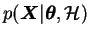is maximised .

The MAP estimate is troublesome because especially in high dimensional spaces, high probability density does not necessarily have anything to do with high probability mass, which is the quantity of interest. A narrow spike can have very high density, but because of its very small width, the actual probability of the studied parameter belonging to it is small. In high dimensional spaces the width of the mode is much more important than its height.

As an example, let us consider a simple linear model for data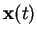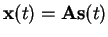(3.8)

where both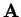and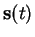are unknown. This is the generative model for standard PCA and ICA .

Assuming that bothandhave unimodal prior distributions centered at the origin, the MAP solution will typically give very small values forand very large values for. This is because there are so many more parameters inthan inthat it pays off to make the sources very close to their prior most probable value, even at the cost ofhaving huge values. Of course such a solution cannot make any sense, because the source values must be specified very precisely in order to describe the data. In simple linear models such behaviour can be suppressed by restricting the values ofsuitably. In more complex models there usually is no way to restrict the values of the parameters and using better approximation methods is essential.

The same problem in a two dimensional case is illustrated in Figure 3.1.3.1 The mean of the two dimensional distribution in the figure lies near the centre of the square where most of the probability mass is concentrated. The narrow spike has high density but it is not very massive. Using a gradient based algorithm to find the maximum of the distribution (MAP estimate) would inevitably lead to the top of the spike. The situation of the figure may not look that bad but the problem gets much worse when the dimensionality is higher.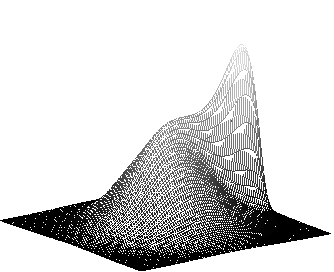SubsectionsNext: Model selection Up: Bayesian methods for data Previous: Conjugate priors   Contents
Antti Honkela 2001-05-30